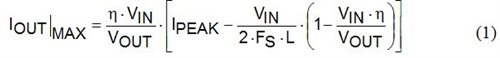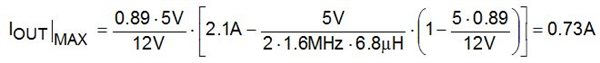# DC/DC converter datasheets- current limit - part two

Other Parts Discussed in Post: LMR62421

In part 1 of this two-part series, I talked about the maximum output current of a step-down or buck DC/DC converter. In this installment, I will look at the step-up or boost converter. Calculating the maximum output current for a boost regulator is somewhat more involved, but still straightforward.

The first thing to understand about a boost converter is that the average inductor current is not equal to the output current, as it is in a buck converter. A boost regulator will still control the inductor current, but this represents the input current of the converter, not the output current. Thus, boost converters are usually specified with a maximum MOSFET current rather than a maximum output current.

As an example, the LMR62421 is called a “2.1A step-up voltage regulator.” This refers to the MOSFET switch current and not the output current. You can use Equation 1 to estimate the maximum output current for a boost converter:First, you need to estimate the efficiency of the converter, η, by looking at the efficiency curves in the data sheet and finding one close to the conditions required in your application. Let’s take an example from the LMR62421 data sheet and find the maximum output current when converting 5V to 12V, using info from the data sheet (Figure 1).Figure 1: Datasheet excerpts for LMR62421

Filling in equation 1 with the data in figure 1 and our input and output conditions, we can calculate the maximum output current:Does this seem like the wrong result? Does it really say that you can only get 0.73A from a “2.1A” boost converter? Yes – and it makes no difference what boost converter you use.

It is not hard to understand this result. For any DC/DC converter, the input and output power will be nearly equal. If your output voltage is higher than the input, then the input current must be higher than the output current, by about the ratio of VOUT/VIN, to give equal power on both sides. Since the MOSFET sees the input current, its rating must be much larger than the required output current. Equation 1 says the same thing mathematically, while taking into account the efficiency of the converter.

Because it is a little tricky to find the maximum output current for a given boost regulator, or to find a boost with a given output current, using the TI WEBENCH® Power Designer tool is the best approach to the problem. The WEBENCH tool will make all of the calculations for you and find a range of suitable converters that match your requirements.